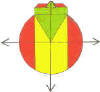DMD   EDU-HOME

WELCOME TO THIS PAGE INTENDED FOR STUDENTS AT THE UNIVERSITY OF THE WESTERN CAPE REGISTERED  FOR THE MODULE:

W4 L3

PROBLEM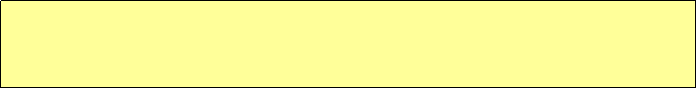An accountant saved 75% of an amount she needs for a new car which she intends to buy cash. She saved on the average R600 per month. If her savings thus far represent 60% of the total saving period (i.e. the total time in which she saves for the required amount), how much must she save in each of the remaining months?

KEY CONCEPTS:

• Percentages
• Money
• Time: Months

DIAGRAM

AMOUNT   A                                                                      TOTAL  T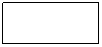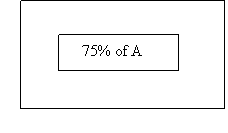= R600 x  (0.6xT)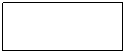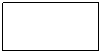= R …..x (0.4xT)

ANALYSIS:

KEY QUESTIONS: HELPS WITH UNDERSTANDING OF PROBLEM

What is the amount required?

How much have you saved?

What are you given? What does this mean/ How can you use it?

What must you find?

SEARCH FOR SOLUTION:  EXPLORE/ WEIGH UP OPTIONS / DECIDE WHAT IS RELEVANT / THINK ABOUT SIMILAR PROBLEMS and their SOLUTIONS.

EXTRACT RELEVANT KNOWLEDGE TO SOLVE PROBLEM:

Do you need to work with ALGEBRAIC EXPRESSIONS / EQUATIONS?  YES/NO

CONSTRUCT the relevant EXPRESSIONS / EQUATIONS

SOLUTION

There are TWO CRITICAL AMOUNTS YOU DON’T KNOW. THEREFORE YOU WILL HAVE TO WORK WITH TWO UNKNOWNS A and T. CENTRAL TO THE SOLUTION IS AN UNDERSTANDING OF BASIC ALGEBRA, the WORKING AND SOLUTION OF LINEAR EQUATIONS

Let A represent the AMOUNT REQUIRED

Therefore, the accountant already saved: ………………………….

Let T be equal to the TOTAL SAVINGS PERIOD.

Now if you saved R600 of 60% of the total saving period T, then you have saved: ……………………………………………

You therefore still require ……………………………….

Thus :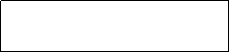0.75 × A = 0.60 ×  T × R600                    ………………………..I

and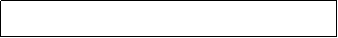0.25 × A = 0.4 × T × AMOUNT REQUIRED       ………. ………II

THUS:

AMOUNT REQUIREDBASIC ALGEBRA:

MAKE A THE SUBJECT OF THE FORMULA in

0.75 × A = 0.60 ×  T × R600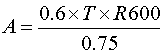(DIVIDE BY 0.75)

RULES FOR EQUATIONS:

1. WHEN REMOVING A TERM FROM ONE SIDE OF AN EQUATION……
2. WHEN REMOVING A FACTOR ……………….

______________________________________________________________

A.                BODMAS (SECTION 2.5, p.77, 147)

B.                ALGORITHM FOR RECURRING DEC. FRACTIONS (CF FORMULA)

C.                DIVISIBILITY TESTS FOR:

• TWO, FOUR, EIGHT
• THREE, NINE
• FIVE
• TEN
• ELEVEN

SIMPLE SIMON: ONE?

BRIGHT SPARKIE: SIX & SEVEN?

D.      NUMBER PATTERNS

E.      INTEGERS

F.       TABLE: p.83

©  DESMOND DESAI, DMD EDU-HOME, 2010

All rights reserved. Designed and created by Desmond Desai, South Africa. This page is protected by Copyright. No part of this page may be reproduced, stored in a retrieval system, or transmitted in any form of by any means, electronic, mechanical, photocopying, recording or otherwise, without the prior written permission of the copyright holder.